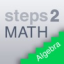Hlavní navigace

#steps2MATH 2.0.0

No more "stupid math" and welcome to steps2Math. Our motto: "Understanding rather than despair." The math app gives students, parents, teachers from grades 5-11 easy access to mathematics. steps2Math solves not only math tasks. It shows and explains the individual steps in the corresponding solutions.

The functions of Steps2MATH:
• Algebra exercises can be entered.
• The solution is generated and displayed.
• For each transformation, there is an explanation.
• The transformations are shown in red and linked to the explanations.
• auxiliary calculation(s) (Addition, Division, Multiplication)
• Function graphs.
• Graphs for both sides of an equation.
• The graphics output includes a table of values.
• The graphs can be scaled and moved.
• For equations, the proof is carried out.
• 35 languages ​​are supported
• They can be switched at any time in the application.
• In some languages​​, the numbers can be displayed in the local representation.
• The application can be adapted to the needs and capabilities of the user.
• An extensive collection of exercises with more than 500 templates.
• The exercises are created again and again.
• Therefore Steps2MATH includes countless exercises.
• Calculations with exact numbers.
• Rounded values, if necessary.
• The exercises can be stored as documents.
• History
• Conversion and transformation of measurement units
• SI units: ampere ... Weber.
• Other units such as inches and yards.

The app can also be used as a scientific calculator.

Mathematical functions:
• Natural numbers
• Integers
• Fractions
• Decimal numbers
• Rational Numbers
• Percentage
• Exponentiation
• forming operations
• Linear equation
• Systems of linear equations
• Roots
• trigonometric functions (π/°)
• Exponential functions
• Logarithm
• Complex numbers
• Sign function
• Roundings
• Absolute value
• Binomial coefficients
• Differential calculus

## Celkové hodnocení

Průměr hodnocení
3

Pro hodnocení programu se prosím nejprve

## Souhrnné informace o steps2MATH

2.0.0

ano

14,96 MB

• #### Jazyk

• Angličtina
• ,
• Čeština
• ,
• Čínština
• ,
• Dánština
• ,
• Egyptština
• ,
• Estonština
• ,
• Finština
• ,
• Francouzština
• ,
• Holandština
• ,
• Italština
• ,
• Japonština
• ,
• Korejština
• ,
• Maďarština
• ,
• Němčina
• ,
• Polština
• ,
• Portugalština
• ,
• Rumunština
• ,
• Ruština
• ,
• Řečtina
• ,
• Španělština
• ,
• Švédština
• ,
• Turečtina
• ,
• Ukrajinština
• ,
• Vietnamština
• #### Staženo

0× celkem
0× tento měsíc

9. 10. 2019

## Něco jsme propásli?

Dejte nám vědět. Upozornit redakci Stahuj
Velice děkujeme za Vaše podněty